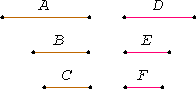# Proposition 20

If there are three magnitudes, and others equal to them in multitude, which taken two and two are in the same ratio, and if ex aequali the first is greater than the third, then the fourth is also greater than the sixth; if equal, equal, and; if less, less.

Let there be three magnitudes A, B, and C, and others D, E, and F equal to them in multitude, which taken two and two are in the same ratio, so that A is to B as D is to E, and B is to C as E is to F.

Let A be greater than C ex aequali.

I say that D is also greater than F; if A equals C, equal; and, if less, less.V.8

Since A is greater than C, and B is some other magnitude, and the greater has to the same a greater ratio than the less has, therefore A has to B a greater ratio than C has to B.

But A is to B as D is to E, and, C is to B, inversely, as F is to E, therefore D has to E a greater ratio than F has to E.

V.10

But, of magnitudes which have a ratio to the same, that which has a greater ratio is greater, therefore D is greater than F.

Similarly we can prove that, if A equals C, then D also equals F, and if less, less.

Therefore, if there are three magnitudes, and others equal to them in multitude, which taken two and two are in the same ratio, and if ex aequali the first is greater than the third, then the fourth is also greater than the sixth; if equal, equal, and; if less, less.

Q.E.D.

## Guide

The statement says that given proportions, a : b =d : e and b : c =e : f, then a >=< c implies d >=< f. The proof is straightforward.

This proposition is in preparation for V.22.